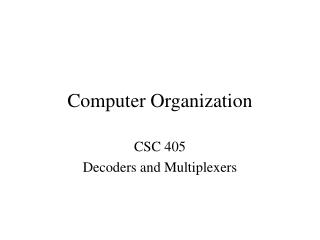DownloadDownload PresentationComputer Organization

# Computer Organization

Download Presentation## Computer Organization

- - - - - - - - - - - - - - - - - - - - - - - - - - - E N D - - - - - - - - - - - - - - - - - - - - - - - - - - -
##### Presentation Transcript

1. Computer Organization CSC 405 Decoders and Multiplexers

2. c3 c2 c1 0 1 2 3 4 5 6 7 3 to 8 decoder Decoders A decoder is a combinatorial circuit with a number of output lines, only one of which is active at any time, depending on the pattern of input lines. A decoder has n control lines to permit the activation of 2n output lines. For example the 3-to-8 decoder shown here allows the designer to activate one of 8 output lines (0 through 7) by applying the appropriate code on the input lines c1, c2 and c3. Any combinatorial circuit of n inputs (i.e. any n-variable Boolean expression) can be represented with an n-to-2n decoder and supporting circuitry.

3. Example: Use a 3-to-8 decoder to build an equivalent circuit the following expression, F(a,b,c) = abc + a’b’ + bc’ a b c abc -> 111 -> m(7) a’b’-> 00- -> m(0,1) bc’ -> -10 -> m(2,6) F(abc) -> m(0,1,2,6,7) F 3 to 8 decoder Homework: Use a 3-to-8 decoder to build an equivalent circuit for the following expression, F(a,b,c)= abc’ + ab’c + a’bc + abc

4. Multiplexers Another combinatorial circuit commonly used in digital design is the multiplexer or selector circuit. This circuit allows you to select one of the inputs to be presented at the output. s1 s0 Control Lines Data Output f 4-to-1 MUX d0 d1 d2 d3 Data Input In general a 2n-to-1 multiplexer selects one of 2n input lines to be passed through to the output line. As with the decoder, the multiplexer can be used to represent any combinatorial circuit.

5. a b c f 0 0 0 1 0 0 1 0 0 1 0 0 0 1 1 1 1 0 0 1 1 0 1 1 1 1 0 0 1 1 1 0 c a b 4-to-1 MUX 1 0 Example: Use an 4-to-1 multiplexer to represent the following Boolean expression, F(a,b,c) = m(0,3,4,5) We will let a and b be the control lines for the MUX. Input c will be distributed across the 4 input lines according to the functional relationships between c and f(a,b,c) implied in the truth table. When a and b are false .................... f=c’ When a is false and b is true then .... f=c When a is true and b is false then .... f=1 When a and b are true then .............. f=0 F

6. Homework: Use a 4-to-1 multiplexer to build the equivalent circuit for the following Boolean expression, F(a,b,c) = m(0,1,2,5,7) What kind of multiplexer could you use to build the equivalent circuit for an expression of four variables, such as, G(p,q,r,s) = m(0,2,5,6,9,11,12,15) ? How could you use a 4-to-1 multiplexer to represent the function G?